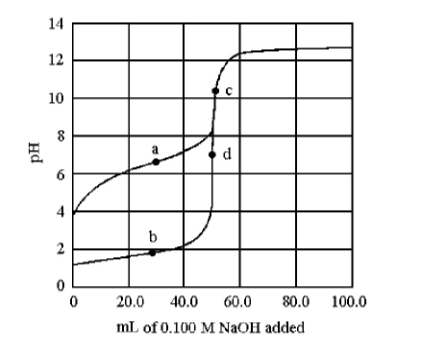# The following plot shows two titration curves, each representing the titration of 50.00 mL of a 0.100 M acid solution with 0.100 M NaOH. (1) Which point represents the equivalence point for the titration of the weaker acid, and (2) which point represents a buffer region?